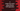# C program to swap two numbers using bitwise XOR operation### C program to swap two numbers using bitwise XOR operation :

In this tutorial, we will learn how to swap two numbers in C using bitwise XOR operation. Let’s take a look at the program :

### C program :

``````#include

int main(){
int firstNumber;
int secondNumber;

printf("Enter the first number : ");
scanf("%d",&firstNumber);

printf("Enter the second number : ");
scanf("%d",&secondNumber);

printf("First number : %d , Second number : %d\n",firstNumber,secondNumber);

firstNumber = firstNumber ^ secondNumber;
secondNumber = firstNumber ^ secondNumber;
firstNumber = firstNumber ^ secondNumber;

printf("After swapping, First number : %d , Second number : %d\n",firstNumber,secondNumber);
}``````

### Explanation :

Let’s try to understand the above program with an example. For firstNumber = 9 and secondNumber = 10, we will calculate the XOR of both . It will become :

``````firstNumber(9)   : 00001001
secondNumber(10) : 00001010

firstNumber = firstNumber XOR secondNumber = 00000011
secondNumber = firstNumber XOR secondNumber = 00000011 XOR 00001010 = 00001001
firstNumber = firstNumber XOR secondNumber = 00000011 XOR 00001001 = 00001010``````

In the above example, you can see that the firstNumber become secondNumber and secondNumber becomes firstNumber .

### Sample Output :

``````Enter the first number : 12
Enter the second number : 14
First number : 12 , Second number : 14
After swapping, First number : 14 , Second number : 12

Enter the first number : 10
Enter the second number : 20
First number : 10 , Second number : 20
After swapping, First number : 20 , Second number : 10

Enter the first number : 3
Enter the second number : 100
First number : 3 , Second number : 100
After swapping, First number : 100 , Second number : 3``````